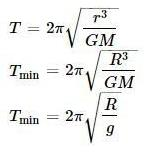Courses

# Test: Gravitation

## 24 Questions MCQ Test Physics Class 11 | Test: Gravitation

Description
This mock test of Test: Gravitation for Class 11 helps you for every Class 11 entrance exam. This contains 24 Multiple Choice Questions for Class 11 Test: Gravitation (mcq) to study with solutions a complete question bank. The solved questions answers in this Test: Gravitation quiz give you a good mix of easy questions and tough questions. Class 11 students definitely take this Test: Gravitation exercise for a better result in the exam. You can find other Test: Gravitation extra questions, long questions & short questions for Class 11 on EduRev as well by searching above.
QUESTION: 1

Solution:
QUESTION: 2

### A man of mass m starts falling towards a planet of mass M and radius R. As he reaches near to the surface, he realizes that he will pass through a small hole in the planet. As he enters the hole, he sees that the planet is really made of two pieces a spherical shell of negligible thickness of mass 2M/3 and a point mass M/3 at the centre. Change in the force of gravity experienced by the man is

Solution:

Gravity does not depend upon shape and size of the celestial body. It depends only on the mass of the two bodies and distance between them.
Fold = GmM/R2 and Fnew = GmM/R2

QUESTION: 3

### A spherical uniform planet is rotating about its axis. The velocity of a point on its equator is V. Due to the rotation of planet about its axis the acceleration due to gravity g at equator is 1/2 of g at poles. The escape velocity of a particle on the pole of planet in terms of V.

Solution: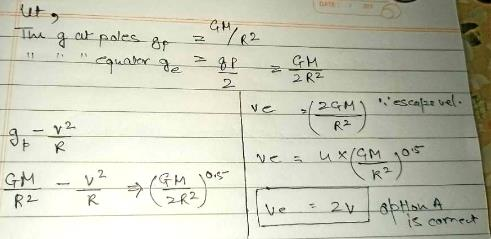QUESTION: 4

The escape velocity for a planet is ve. A tunnel is dug along a diameter of the planet and a small body is dropped into it at the surface. When the body reaches the centre of the planet, its speed will be

Solution: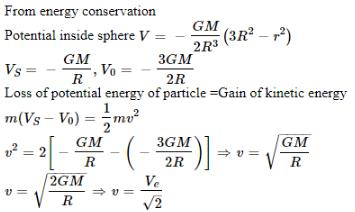QUESTION: 5

If a tunnel is cut at any orientation through earth, then a ball released from one end will reach the other end in time (neglect earth rotation)

Solution:

Total time period will be 84.6 min when ball released from one end and it come backs to same point as in oscillation.
When ball is released from one end then time taken to reach other end will be half of total time period then that will be 42.3 min.

QUESTION: 6

Two uniform spherical stars made of same material have radii R and 2R. Mass of the smaller planet is m. They start moving from rest towards each other from a large distance under mutual force of gravity. The collision between the stars is inelastic with coefficient of restitution 1/2.

Kinetic energy of the system just after the collision is

Solution: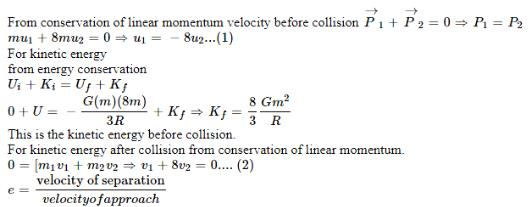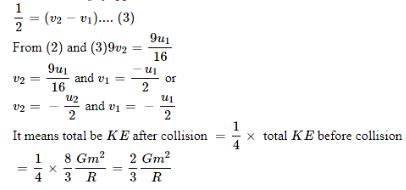QUESTION: 7

Two uniform spherical stars made of same material have radii R and 2R. Mass of the smaller planet is m. They start moving from rest towards each other from a large distance under mutual force of gravity. The collision between the stars is inelastic with coefficient of restitution 1/2.

The maximum separation between their centres after their first collision

Solution:
QUESTION: 8

A mass is at the center of a square, with four masses at the corners as shown.

(A)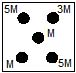(B)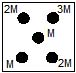(C)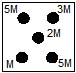(D)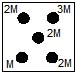Rank the choices according to the magnitude of the gravitational force on the center mass.

Solution:

Gravitational force by mass M on another body of m at a distance of r is given by F=GMmr2
For given figure Right diagonal is x axis and left y axis in figure A forces due to 5M will cancel out each other so effective force will be due to only 3M an M. In figure B 2M forces will cancel out each other and effective force will be due to 3M and M.
Hence total force on M in both the cases will be the same.
Similarly, in figure C, 5M mass will cancel each other out and in Figure D, 2M will cancel each other out and effective force will be due to 3M and M on the mass of 2M. So, force on c and d will be the same.
Force on C will be more than B.
Hence answer is FA​=FB​<FC​=FD​

QUESTION: 9

A satellite of mass m, initially at rest on the earth, is launched into a circular orbit at a height equal to the radius of the earth. The minimum energy required is

Solution:
QUESTION: 10

A satellite of mass 5M orbits the earth in a circular orbit. At one point in its orbit, the satellite explodes into two pieces, one of mass M and the other of mass 4M. After the explosion the mass M ends up travelling in the same circular orbit, but in opposite direction. After explosion the mass 4M is in

Solution:

Applying the conservation of momentum, we get:
V = velocity of 5M, V1 = velocity of 4M, V2 = velocity of M and ATQ V2 = -V
5MV = 4MV1 + M(-V)
6MV = 4MV1
V1 = 3/2 V
Now, Vo = orbital velocity and Ve = escape velocity
Ve = √2 Vo
In a bound orbit the object is gravitationally bound to the body that is the source of gravity (like the Earth is bound to the Sun, or the Moon to the Earth). An unbound orbit is typically hyperbolic, and the object will escape from the source of gravity

QUESTION: 11

The orbit of a planet P round the sun S. AB and CD are the minor and major axes of the ellipse.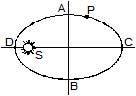If t1 is the time taken by the planet to travel along ACB and t2 the time along BDA, then

Solution:

Here, the angular momentum is conserved i.e. mvr is constant. Where r is the distance from centre of the sun to centre of the planet.
Now, consider one point each from both the mentioned paths. (Shown in fig.)
Applying conservation of angular momentum for these points we get
mv1​r1​=mv2​r2​. Simplifying this, v1/​v2​​=r2​/r1​​<1
Time period are given by
t1​=L/ v1​, t2​= L​/ v2
Comparing them by taking the ratio
t1/t2​​=(L​/ v1)×(v2/L)
So, t1/t2=v2​/v1​​>1.Thus t1​>t2

QUESTION: 12

The orbit of a planet P round the sun S. AB and CD are the minor and major axes of the ellipse.If U is the potential energy and K kinetic energy then |U| > |K| at

Solution:

If a planet is orbiting the sun then |U|> |K| else it will leave the system because it won’t be under the influence of the sun’s gravity.

QUESTION: 13

Satellites A and B are orbiting around the earth in orbits of ratio R and 4R respectively. The ratio of their areal velocities is

Solution:

Answer :- a

Solution :- L = mvr

⇒L=m√GMr/r

L = m√GMr−−−−.....(1)

L = 2mdA/dt....(2)

From (1) and (2)

dA/dt ∝ (r)^1/2

= √(dA/dt)1/(dA/dt)2

= √4/1 = 2/1

QUESTION: 14

Figure shows the variation of energy with the orbit radius r of a satellite in a circular motion. Select the correct statement.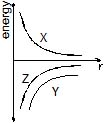Solution:

Kinetic Energy of the Satellite =GMm​/2r
Potential Energy of the satellite =−GMm​/r
Total Energy of the Satellite =−GMm​/2r
Hence, Kinetic Energy Cure will be above 0 , hence X
Potential energy will be the lowest curve , hence Y
Total Energy is the curve is below 0 , but higher magnitude than Potential Energy  . Hence, Z

*Multiple options can be correct
QUESTION: 15

Assuming the earth to be a sphere of uniform density the acceleration due to gravity

Solution:

As g is inversely proportional to (R+h)2 when we go away from earth's surface g is inversely proportional to square of the distance and g is directly proportional to (R-d) when we go inside the surface of Earth therefore g is directly proportional to distance travelled inside the Earth
g=Gmr/a3=r for r<R
g=Gm/a2 for r<R

*Multiple options can be correct
QUESTION: 16

Two masses m1 and m2 (m1 < m2) are released from rest from a finite distance. They start under their mutual gravitational attraction

Solution: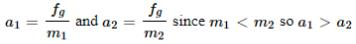The total energy of the system remains constant.

*Multiple options can be correct
QUESTION: 17

In side a hollow spherical shell

Solution:

The gravitational field inside a uniform spherical shell is 0 from gauss law for gravitation since no mass is enclosed in any Gaussian surface.
Since gravitational potential is given by φ=−∫ gdr, hence, φ=constant since g=0.
Since the gravitational field is 0 everywhere, it is apparently the same everywhere.
Answer is B,C,D.

*Multiple options can be correct
QUESTION: 18

A geostationary satellite is at a height h above the surface of earth. If earth radius is R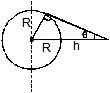Solution:

Minimum colatitude=sin θ=R/R+h
θ=sin-1(R/R+h)
The curved area AB on earth =2πR2(1−sinθ)
Area on earth escaped from satellite =4πR2−2πR2(1−sinθ)
=2πR2(1+sinθ)

*Multiple options can be correct
QUESTION: 19

When a satellite in a circular orbit around the earth enters the atmospheric region, it encounters small air resistance to its motion. Then

Solution:

Due to air drag some mechanical energy of satellite will converted into heat energy, there will be loss of ME of satellite, so radius of orbit will decrease and satellite will follow a spiral path towards earth.
If r is decreases,
P.E.=−GMm​/r, P.E. is also decreases.
K.E=GMm​/2r K.E increasing, increased speed in spite of decrease in M.E. But rate of P.E decreases is more than the rate at which M.E decreases.
Angular momentum L=mrv=mr√GM​r=m√GMr​⇒L∝√r​.
So, angular momentum decreases as r decreases.

*Multiple options can be correct
QUESTION: 20

A communications Earth satellite

Solution:

Communication satellites are supposed to be geostationary in nature. So, from the above properties options B is correct.

*Multiple options can be correct
QUESTION: 21

An earth satellite is moved from one stable circular orbit to another larger and stable circular orbit. The following quantities increase for the satellite as a result of this change

Solution:

Let’s see the formulae for each quantity given there,
Gravitational potential energy  =−GMm/r​
angular velocity =(GM/r3​)0.5
linear orbital velocity  =(GM/r​)0.5
centripetal acceleration  =GM/ r2
We can observe that, the quantities in the options B,C,D are all indirectly proportional to r
i.e. ω, v, a α(1/rn)​ where n is a distinct positive integer in each quantity.
So, with increase in r all these quantities decrease.
Now, (-potential energy) α(1/r)​ i.e. negative of potential energy decreases with increase in r. Therefore, potential increases with increase in orbital radius.

*Multiple options can be correct
QUESTION: 22

A satellite S is moving in an elliptical orbit around the earth. The mass of the satellite is very small compared to the mass of the earth

Solution:

As the gravitational force on satellites due to earth acts always towards the centre of earth, thus acceleration of S is always directed towards the centre of the earth.
Also, as there is no external force so according to conservation of energy, total mechanical energy of S is constant always.
Also, as in the absence of external torque L is constant in magnitude and direction.
Thus, mrv=constant ⟹v varies as r changes
Hence, p=mv is not constant.

*Multiple options can be correct
QUESTION: 23

If a satellite orbits as close to the earth's surface as possible,

Solution:

The velocity of satellite V=√GM​/R​⇒V∝1/√R​​.
The time period of satellite is given by  T=2π√ R3/GM​​⇒T∝R1.5
The total energy is given by  T.E.=−GMm/2R​⇒T.E.∝−1/M​
Here, G is constant, R is orbital radius, M is mass of earth and m is mass of satellite.
Near the earth surface, R has the least value and therefore time period and total energy are minimum while velocity is maximum. So, options A, B, C are correct.

*Multiple options can be correct
QUESTION: 24

For a satellite to orbit around the earth, which of the following must be true ?

Solution: